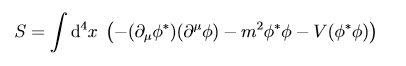# Complex scalar field, conserved current, expanding functional

## Homework Statement

[/B]
Hi

I am looking at this action:

Under the transformation ## \phi \to \phi e^{i \epsilon} #### Homework Equations

[/B]
So a conserved current is found by, promoting the parameter describing the transformation- ##\epsilon## say- to depend on ##x## since we know that ##\delta S=0## on-shell trivially. So we find a quantity that has ##\delta S \neq 0## when ##\epsilon=\epsilon(x)##, but ##\delta S=0## when ##\epsilon## does not depend on ##x##.

I have read that the conserved current is the same as it is without the potential for the addition of a potential ##V(\phi \phi*)## in the Lagrangian.

So it must be that ## \delta V(\phi \phi*) =0 ## for both ##\epsilon## depending on ##x## or not depending on ##x##. Since there are no derivatives this is just that ## \delta V(\phi \phi*) =0 ##, required for the action to be invariant under the transformation anyway.

## The Attempt at a Solution

## V(\phi \phi*) \to V(\phi \phi* e^{i \epsilon} e^{-i \epsilon}= V(\phi \phi*) ##

So looking at it from this it is obvious.

But, my question / point of my post, if I expand out ## V(\phi \phi*)## how do I show this?

I believe I can write: ##V[\phi,\phi*]=V[\phi,\phi*]+\frac{\partial V[\phi,\phi*]}{\partial \phi} \delta\phi + \frac{\partial V[\phi,\phi*]}{\partial \phi*} \delta\phi *  ##

??

in this case I know that ##\delta\phi=-\delta\phi* ## to ##O(\epsilon)=O(\delta)##

Therefore looking at  I see that it must be that ##\frac{\partial V[\phi,\phi*]}{\partial \phi} \delta\phi + \frac{\partial V[\phi,\phi*]}{\partial \phi*} \delta\phi *=0##

##=-\frac{\partial V[\phi,\phi*]}{\partial \phi} \delta\phi* + \frac{\partial V[\phi,\phi*]}{\partial \phi*} \delta\phi *##

so it must be that ##\frac{\partial V[\phi,\phi*]}{\partial \phi}=\frac{\partial V[\phi,\phi*]}{\partial \phi*} ##

Is this true because rather than ##V[\phi,\phi*] ## we have ##V[\phi \phi*] ##; the potential is symmetric in both ##phi## and ##phi*##?

But then I should have a 1 variable taylor expansion rather than 2 variable as I treated it to get ?

How would I expand out something like ##V[\phi\phi* + \delta\phi* \phi + \delta \phi \phi*] ## treating ##\phi\phi*## as a single variable, rather what I have expanded out is ##V[\phi + \delta\phi, \phi* + \delta \phi*]##? (if this is something I've done wrong here, thanks)

#### Attachments

Last edited:
•Delta2

## Homework Statement

[/B]
Hi

I am looking at this action:

Under the transformation ## \phi \to \phi e^{i \epsilon} ##

View attachment 218290

## Homework Equations

[/B]
So a conserved current is found by, promoting the parameter describing the transformation- ##\epsilon## say- to depend on ##x## since we know that ##\delta S=0## on-shell trivially. So we find a quantity that has ##\delta S \neq 0## when ##\epsilon=\epsilon(x)##, but ##\delta S=0## when ##\epsilon## does not depend on ##x##.

I have read that the conserved current is the same as it is without the potential for the addition of a potential ##V(\phi \phi*)## in the Lagrangian.

So it must be that ## \delta V(\phi \phi*) =0 ## for both ##\epsilon## depending on ##x## or not depending on ##x##. Since there are no derivatives this is just that ## \delta V(\phi \phi*) =0 ##, required for the action to be invariant under the transformation anyway.

## The Attempt at a Solution

## V(\phi \phi*) \to V(\phi \phi* e^{i \epsilon} e^{-i \epsilon}= V(\phi \phi*) ##

So looking at it from this it is obvious.

But, my question / point of my post, if I expand out ## V(\phi \phi*)## how do I show this?

I believe I can write: ##V[\phi,\phi*]=V[\phi,\phi*]+\frac{\partial V[\phi,\phi*]}{\partial \phi} \delta\phi + \frac{\partial V[\phi,\phi*]}{\partial \phi*} \delta\phi *  ##

??

in this case I know that ##\delta\phi=-\delta\phi* ## to ##O(\epsilon)=O(\delta)##

Therefore looking at  I see that it must be that ##\frac{\partial V[\phi,\phi*]}{\partial \phi} \delta\phi + \frac{\partial V[\phi,\phi*]}{\partial \phi*} \delta\phi *=0##

##=-\frac{\partial V[\phi,\phi*]}{\partial \phi} \delta\phi* + \frac{\partial V[\phi,\phi*]}{\partial \phi*} \delta\phi *##

so it must be that ##\frac{\partial V[\phi,\phi*]}{\partial \phi}=\frac{\partial V[\phi,\phi*]}{\partial \phi*} ##

Is this true because rather than ##V[\phi,\phi*] ## we have ##V[\phi \phi*] ##; the potential is symmetric in both ##phi## and ##phi*##?

But then I should have a 1 variable taylor expansion rather than 2 variable as I treated it to get ?

How would I expand out something like ##V[\phi\phi* + \delta\phi* \phi + \delta \phi \phi*] ## treating ##\phi\phi*## as a single variable, rather what I have expanded out is ##V[\phi + \delta\phi, \phi* + \delta \phi*]##? (if this is something I've done wrong here, thanks)

thanks for the like @Delta² , I don't suppose you may be able to help at all? thanks alot.

Delta2
Homework Helper
Gold Member
Ah, sorry I have my own doubts/questions about this so I wont be much of help if I say anything.

Always nice to see a post with an action integral invariant under some transform, that's why I gave it a like :D.

•binbagsss